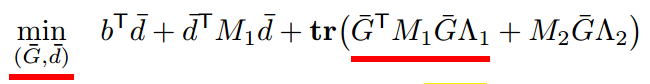# Multiplication of Matrix

I want to solve the below problem:I tried cvx first:

variables G(9,18) d(9)

the other matrix are constant.

G’M1G*Lamb1 will report ‘Only scalar quadratic forms can be specified in CVX’.

But I tried yalmip later and solved this problem ( no non-convex reported).

I wonder G’M1G is a very common expression in optimal problems, why cvx can not handle it?

Thanks!

The reasons are twofold. First of all, CVX is not a symbolic processing engine; it assembles every subexpression as it is constructed. And secondly, every subexpression must obey the ruleset. So CVX sees G^TMG first, which is not DCP compliant, and rejects it.

If M_1 and \Lambda_1 are positive semidefinite, then that first term in the trace is equivalent to `square_pos(norm(sqrtm(M1)*G*sqrtm(L1)))`.

Dear Prof. Grant,